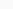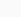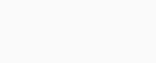# AP 9th Physical Science Mock Test 2021 for Chapter 6 : “What is inside the atom ?” Online Practice Test

1. An alpha particle is a bundle of
2. A proton may be represented by
3. An atom having 10 electrons and 10 neutrons is represented as
4. Oxygen , magnesium , phosphorus and sulphur have atomic numbers 8 , 12 , 15 and 16 respectively , which of these does not posses a valency of 2 ?
5. Outermost shell of an atom cannot accommodate electrons more than
6. Rutherford discovered the
7. Isotopes have
8. Hydrogen nucleus is otherwise called
9. The effective diameter of nucleus  is approximately
10. The discharge tube experiments were first carried by
11. Electron is called as universal particle. The reason is
12. In thisray scattering experiment , Rutherford used a very thin foil made of gold metal.The reason is
13. Two elements A and B have the atomic numbers , 18 and 20 respectively . Choose the correct statement , regarding these elements
14. The wave nature of electrons was given by
15. Find out the wrong statement
16. The size of an atom is decided by
17. Atomic number of chlorine is 17.Which shell is incompletely filled in this atom ?
18. Atoms can achieve an octet by the
19. The first subatomic particles to be discovered and studied are
20. Multiple valency is exhibited by the element
21. The number electrons in F is
22. Rutherford's model of an atom is called
23. Electrons are found only in certain energy levels called
24. The number of electrons present in the outermost orbit of an atom is called
25. The total number of neutrons and protons in the nucleus is called
26. The property of having 8 electrons in the outer most orbit
27. The number of outermost electrons present in Na+ ion is
28. An atom with 3 protons and 4 neutrons has the atomic number
29. The maximum number of electrons that can be accommodated in'L' shell is
30. The shell closest to the nucleolus has............. energy
31. The standard notation of an atom is. the option which shoes oxygen atom is
32. Dippi : By transfer of electrons atoms - can attain octet
Beli : By sharing of electrons atoms - can attain octet
33. Atoms are considered electrically
34.- particles scattering experiment helped to detect the existence of the nucleus. The main observations of this experiment is
35.- particles scattering experiment confirm
1. Nucleus is positively charged
2. Nucleus contains electrons
3. Electrons revolve around the nucleus
4. Most of the space inside the atom is empty
36. Which is fundamental particle of atom...............
37. Which of the following is the set of Isotopes ...........
38. Based on scientists and their inventions match following
1. Bohr - Bury           ( ) a. Existence of a nucleus
2. Thompson             ( ) b. Maximum no.of electrons in a shell
3. Rutherford            ( ) c. subatomic particle
39. Match the following

P. Atomic number            ( ) X. No.of protons
Q. Atomic mass number ( ) Y. A - Z
R. Number of neutrons   ( ) Z. No.of Protons + No.of neutrons
40. The distribution of electrons in the above diagram represents the element41. The symbol '+' represents
42. The first model of atom was suggested by
43. If we use iodized salt in our daily life we can be prevented by the disease
44. The mass of an electron is nearly equal to this part of the mass of a proton
45. Isotopes are used in the laboratory to investigate the steps of chemical reactions and medical mysteries . An isotope which is used in the treatment of Goitre is
46. The shell which has lowest energy in an atom is
47. The number of neutrons in92U238 ........
48. Electronic configuration of fluorine is
49. The valency of argon is
50. The no.of protons and no.of neutrons is called mass number . The no.of neutrons present in the sodium atom whose atomic number is
51. Arrangement of electrons in an atom with atomic number = 5 and valency = 3 is
52. Application of isotopes are
53. During the electrolysis of experiment ..................... discovered the atoms were acquiring negative charge during the process of electrolysis
54. Faraday discovered that atoms were acquiring the .......... charge during process of electrolysis
55. The particles smaller than the atom and present inside the atom is called
56. The particle which has a negatively charged present in atom and having the very small mass is called as
57. Electron is represented as
58. If an atom has only electrons to neutralize them we should have
59. The particle which neutral the atom which has electron is
60. The mass of proton is approximately ............ more than the electron
61. Proton is represented as
62. Neutron is represented as
63. The particle which mass is nearly equal to proton and have no charge is
64. Atom is made up of
65. Position of electron is
66. Which particles present inside the atom ?
67. Plum Pudding Model is proposed by
68. J.J Thomson proposed Plum Pudding Model in
69. The following is a70. In the following diagram the black seeds represent the71. Alpha particles scattering experiment using gold foil is done by
72. The following was done in73. The following was explained74. Positively charged particles in an atom formed a small dense centre , called
75. S : Nucleus is very small
T : Atom has larger size than nucleus
76. Which of the following is not a Limitations of Rutherford's atomic model ?
77. Which of the following are postulates about model of an atom ?
78. The electrons revolves round the nucleus in certain , discrete circular orbits of the atom , these orbits or shell are called
79. In the following the letters K , L , M , N are80. Shell number is represented as
81. Electrons in different shells have
82. The shell closest to the nucleus is called
83. K-Shell has higher energy than
84. The maximum number of electrons in N-shell is
85. The maximum number of electrons in K-shell is
86. According to................ there should be 2 electrons in innermost shell
87. The number of electrons present in outermost orbit of an atom is called
88. Carbon valency
89. Valency of Sulphur
90. Number electron present in Argon is
91. No.of protons present in Hydrogen
92. Match the following based on atomic number
1. Hydrogen    ( ) a. He
2. Helium        ( ) b. Li
3. Lithium       ( ) c. H
93. An atom can achieve an octet by
94. Number of protons in a nucleus is denoted by
95. Atomic mass number =
96. In the formula A = Z + N , N represents
97. The number of nucleons , the total number of protons and neutrons in an atom is called
98. Elements differ from one another according to the number of protons in their atomic nuclei . This value is called
99. ............... number is number of protons in the nucleus of an atom
100. Match the following based on valencies
1. Neon         ( ) a. 4
2. Carbon     ( ) b. 0
3. Fluorine   ( ) c. 3
4. Boran        ( ) d. 1